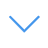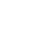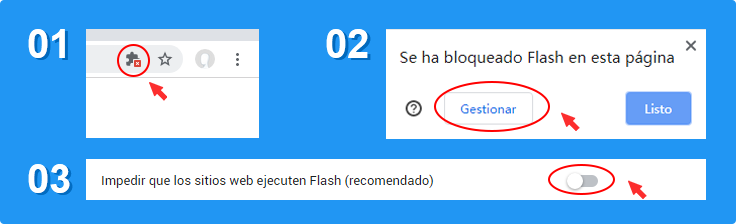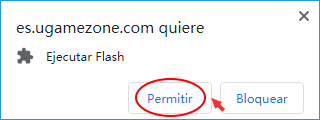# Solve Math

Solve Math is one of the Math Games that you can play on UGameZone.com for free. Are you sensitive to mathematical calculations? This game challenges the player's responsiveness and mathematical computing skills. You have to solve the equals answer by given random numbers and mathematical symbols.

Read MorePresiona 【Esc】 para salir de pantalla completa

# Solve Math

Solve Math is one of the Math Games that you can play on UGameZone.com for free. Are you sensitive to mathematical calculations? This game challenges the player's responsiveness and mathematical computing skills. You have to solve the equals answer by given random numbers and mathematical symbols.Para jugar el juego, es necesario para permitir Adobe Flash Player para ejecutarse en su navegador.

Puede usar el complemento UGameZone Flash Enabler para habilitar Flash automáticamente. O puede seguir sus instrucciones para habilitar Flash manualmente.

Juega automáticamente

## Game Ratings

75%

Teatraĵoj 500

Voĉoj 700

700
0
Category
Math Games
Etikedoj
Priskribo

Solve Math is one of the Math Games that you can play on UGameZone.com for free. Are you sensitive to mathematical calculations? This game challenges the player's responsiveness and mathematical computing skills. You have to solve the equals answer by given random numbers and mathematical symbols.

Paso 1
Paso 2
Habilite Adobe Flash Player para jugar "Solve Math"

Habilitar Flash en la configuración de Chrome. hacer clic "Bloquear sitios para que no ejecuten Flash " cambiar a " Pregunta primero ".Mostrado como el siguiente:Ahora haz clic en y haz clic en el botón " Permitir " en la esquina superior izquierda de Chrome para jugar. Mostrado como el siguiente: